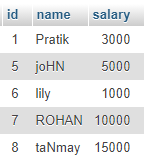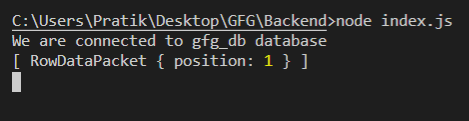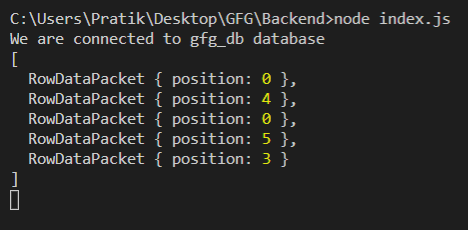GFG App
Open AppBrowser
Continue

# Node.js MySQL INSTR() Function

INSTR() function is a built-in function in MySQL that is used to get the position of the first occurrence of pattern in the text.

Note: In NodeJs MySQL, INSTR() function is not case sensitive.

Syntax:

`INSTR(text, pattern)`

Parameters: It takes two parameters as follows:

• text: It is the given text in which the pattern is searched.
• pattern: It is the pattern which the user wants to search in the text.

Return Value: It returns a position number of the first occurrence of pattern in the text. If occurrence not found then it will return 0.

Module Installation: Install the mysql module using the following command:

`npm install mysql`

Database: Our SQL publishers table preview with sample data is shown below:Example 1:

## index.js

 `const mysql = require(``"mysql"``); ` ` `  `let db_con  = mysql.createConnection({ ` `    ``host: ``"localhost"``, ` `    ``user: ``"root"``, ` `    ``password: ``''``, ` `    ``database: ``'gfg_db'` `}); ` ` `  `db_con.connect((err) => { ` `    ``if` `(err) { ` `      ``console.log(``"Database Connection Failed !!!"``, err); ` `      ``return``; ` `    ``} ` ` `  `    ``console.log(``"We are connected to gfg_db database"``); ` ` `  `    ``// Here is the query ` `    ``let query =  ` `"SELECT INSTR('GeeksForGeeks', 'geek') AS position"``; ` ` `  `    ``db_con.query(query, (err, rows) => { ` `        ``if``(err) ``throw` `err; ` ` `  `        ``console.log(rows); ` `    ``}); ` `});`

Run the index.js file using the following command:

`node index.js`

Output:Example 2:

## index.js

 `const mysql = require(``"mysql"``); ` ` `  `let db_con  = mysql.createConnection({ ` `    ``host: ``"localhost"``, ` `    ``user: ``"root"``, ` `    ``password: ``''``, ` `    ``database: ``'gfg_db'` `}); ` ` `  `db_con.connect((err) => { ` `    ``if` `(err) { ` `      ``console.log(``"Database Connection Failed !!!"``, err); ` `      ``return``; ` `    ``} ` ` `  `    ``console.log(``"We are connected to gfg_db database"``); ` ` `  `    ``// Here is the query ` `    ``let query =  ` `"SELECT INSTR(name, 'n') AS position FROM publishers"``; ` ` `  `    ``db_con.query(query, (err, rows) => { ` `        ``if``(err) ``throw` `err; ` ` `  `        ``console.log(rows); ` `    ``}); ` `});`

Run the index.js file using the following command:

`node index.js`

Output:My Personal Notes arrow_drop_up